# Free Ncert Class 6 Math Mensuration Exercise 10.3

Mensuration Class 6 Ex. 10.3

Mensuration, Ncert Class 6, Chapter 10, Exercise 10.2 Math Free Solution.

Exercise 10.3

Question 1:- Find the areas; of the rectangle whose sides are :-
(a) 3 cm and 4 cm
(b) 12 m and 21 m
(c) 2 km and 3 km
(d) 2 m and 70 cm

Solution 1: –
(a) Length of the rectangle = 3cm
Breadth of the rectangle = 4cm
Since, the area of the rectangle = length x breadth
= 3 cm x 4 cm
= 12 square cm.

(b) Length of the rectangle = 12m
Breadth of the rectangle = 21m
Since, the area of the rectangle = length x breadth
= 12 m x 21 m
= 252 square m.

(c) Length of the rectangle = 2km
Breadth of the rectangle = 3km
Since, the area of the rectangle = length x breadth
= 2 km x 3 km
= 6 square km

(d) Length of the rectangle = 2m
Breadth of the rectangle = 70cm = 0.70m
Since, the area of the rectangle = length x breadth
= 2 m x 0.70 m
= 1.4 square m

Question 2:- Find the areas; of the square whose sides are :-
(a) 10 cm
(b) 14 cm
(c) 5 m

Solution 2:-
(a) The side of the square = 10 cm;
Since, the area of the square = Side x Side;                         [By the formula of area of square]
= 10 cm x 10 cm
= 100 square cm.

(b) The side of the square = 14 cm;
Since, the area of the square = Side x Side;
= 14 cm x 14 cm
= 196 square cm.

(c) The side of the square = 5 cm;
Since, the area of the square = Side x Side;
= 5 m x 5 m
= 25 square m.

Question 3 :- The length and breadth of three-rectangle are as given below :-
(a) 9 m and 6 m
(b) 17 m and 3 m
(c) 4 m and 14 m
Which one has the largest-area and which one has the smallest area?

Solution 3:-
(a) Length of the rectangle = 9m
Breadth of the rectangle = 6m
Since, the area of the rectangle = length x breadth
= 9 m x 6 m
= 54 square m.

(b) Length of the rectangle = 17m
Breadth of the rectangle = 3m
Since, the area of the rectangle = length x breadth
= 17 m x 3 m
= 51 square m.

(c) Length of the rectangle = 4m
Breadth of the rectangle = 14m
Since, the area of the rectangle = length x breadth
= 4 m x 14 m
= 56 square m

The rectangle (c) has the largest area, i.e., 56 square meter and rectangle (b) has the smallest area, i.e., 51 square meter.

Question 4 :- The area; of a rectangular-garden 50m long is 300 square m. Find the width; of the garden.

Solution 4:-
Length of the rectangle garden = 50m
Breadth of the rectangle = 300 square meter
Since, the area of the rectangle = length x breadth
300 = 50 m x breadth
Breadth = 300/50 m = 6 m.

Hence, the breadth of the garden is 6m.

Question 5 :- What is the cost of tiling a rectangular-plot of land 500m long and 200m wide at the rate of ₹8 per hundred square m?

Solution 5:-

Length of the rectangle plot = 500m
Breadth of the rectangle plot = 200m
Since, the area of the rectangle = length x breadth
= 500 m x 200 m
= 1,00,000 square m

Now rate of tiling the plot = ₹8 per 100 square meter
Cost of tiling 100 square meter land = ₹8

Cost of tiling 1,00,000 square meter land = ₹(8/100) x 1,00,000 = ₹8000
Hence the required cost = ₹8000.

Question 6 :- A table-top measures 2m by 1m 50cm. What is its area in square meter?

Solution 6:-
the length of the table-top = 2 m
the breadth of the table-top = 1m 50cm or 1.50 m
Since, the area of the table-top = length x breadth
= 2 m x 1.50 m
= 3 square meter

Hence, the area of table-top = 3 square metre.

Question 7 :- A room is 4m-long and 3m 50cm wide. How many square-metres of carpet is needed to cover the floor of the room?

Solution 7:-
The length of the room is = 4 m
The breadth of the room = 3m 50cm = 3.5 m
Since, the area of the room = length x breadth
= 4 m x 3.5 m = 14 square meter

Hence, the area of the carpet needed = 14 square meter.

Question 8 :- A floor is 5m long and 4m wide. A square-carpet of sides 3m is laid on the floor. Find the area of the floor that is not carpeted ?

Solution 8:-
The length of the floor = 5m
The breadth of the floor = 4 m
Since, the area of the floor = length x breadth
= 5m x 4m = 20 square meter;

Side of the square-carpet = 3m
Since, the area of the square-carpet = side x side
= 3m x 3m = 9 square meter

So, the area of the floor which is not carpeted = 20 sq m – 9 sq m = 11 square meter.

Question 9 :- Five-square; flower beds each of side 1m are dug on a piece of land 5m long and 4m wide. What is the area; of the remaining part of the land?

Solution 9:-
The side of the one square flower-bed = 1 m.
Since, the area of 1 square flower bed = 1m x 1m = 1 square meter.
So, the area of 5 square flower beds = 1 sq m x 5 = 5 square meter.

Now, the length of a piece of land = 5m;
the breadth of the land = 4 m
Since, the area of the land = length x breadth
=5m x 4m = 20 square meter

So, the area of the remaining part of the land = 20 sq m – 5 sq m = 15 square meter.

Question 10 :- By splitting the following figures into rectangle, find their areas ? (The measures are given in centimetres).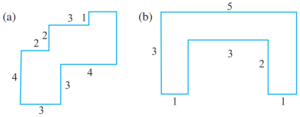Solution 10:-(a) Splitting the given figure into 4 parts

Since, the area of the rectangle = length x breadth;

The area of HKLM = 3 x 3 = 9 square cm
The area of HIJG = 1 x 2 = 2 square cm
The area of GFED = 3 x 3 = 9 square cm
The are of ABCD = 2 x 4 = 8 square cm

So, the total area of the whole figure :-
= 12 sq cm + 6 sq cm + 4 sq cm + 6 sq cm = 28 square cm.

(b) Splitting the given figure into 4 parts, we get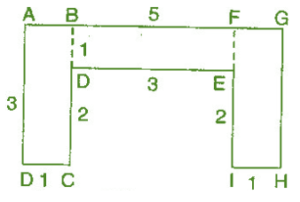The area of ABCD = 3 x 1 = 3 square cm
The area of BFED = 3 x 1 = 3 square cm
The area of FGHL = 3 x 1 = 3 square cm

So, the total area of the given figure :-
= 3 sq cm + 3 sq cm + 3 sq cm = 9 square cm.

Question 11 :- Split the following shape into rectangle and find their area (The measures are given in centimetres).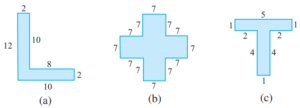Solution 11:-
(a) Splitting the given figure into 2 parts , we get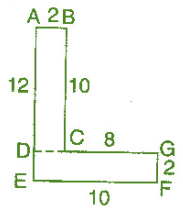Since, the area of the rectangle = length x breadth

The area of ABCD = 10 x 2 = 20 square cm
The are of DGFE = 10 x 2 = 20 square cm

So, the total area of the whole given figure = 20 sq cm + 20 sq cm = 40 square cm.

(b) Splitting the given figure :-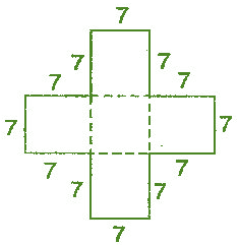Since, the Area of square = side x side
= 7 cm x 7 cm = 49 square cm

Since, the given figure has 5 identical square of side 7 cm

So, the total area of the whole figure
= 49 sq cm + 49 sq cm + 49 sq cm + 49 sq cm + 49 sq cm = 245 sq cm.

(c) Splitting the given figure into 2 parts , we get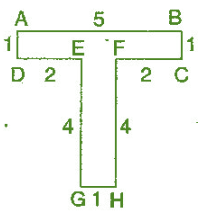Since, the area of the rectangle = length x breadth

The area of ABCD = 5 x 1 = 5 square cm
The are of EGHF = 4 x 1 = 4 square cm

So, the total area of the whole figure = 5 sq cm + 4 sq cm = 9 square cm.

Question 12 :- How many tiles; whose length and breadth are 12cm and 5cm respectively will be needed to fit in a rectangular-region whose length and breadth are respectively : –
(a) 100cm and 144cm;
(b) 70cm and 36cm;

Solution 12:-
Length of the one tile = 12 cm
Breadth of the one tile = 5 cm
Since, the area of the rectangle = length x breadth
= 12 cm x 5 cm = 60 square cm

(a)  The length of the rectangular region = 144 cm
The breadth of the rectangular region = 100 cm
Since, the area of the rectangular region = length x breadth
= 144 cm x 100 cm
= 14,400 square cm

So, the number of tiles needed to cover the whole rectangular region
= 14400 square cm ÷ 60 square cm
= 240 tiles.

(b) The length of the rectangular region = 70 cm
The breadth of the rectangular region = 36 cm
Since, the area of the rectangular region = length x breadth
= 70 cm x 36 cm
= 2,520 square cm

So, the number of tiles needed to cover the whole rectangular region
= 2,520 sq cm ÷ 60 sq cm
= 42 tiles.

1.